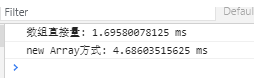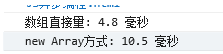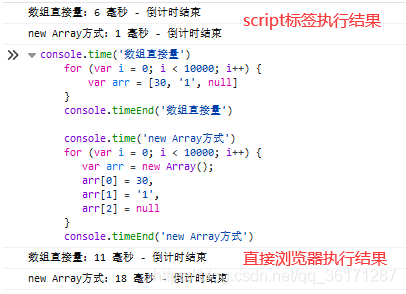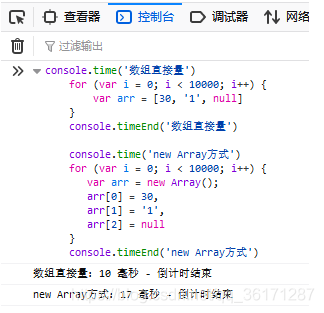# 高性能 JavaScriptの七 -- 编程实践小技巧# 避免双重求值（Double Evaluation）

JavaScript 与很多其他语言一样，允许你在程序中提取一个包含代码的字符串，然后动态执行

• Function() 构造函数

• eval()

• setTimeout()

• setInterval()

var num1 = 1,num2 = 6;//eval()执行代码字符串sum = eval("num1 + num2");//Function()构造函数执行代码字符串sum = new Function("num1", "num2", "return num1 +num2");//setTimeout()执行代码字符串setTimeout("sum = num1 +num2", 100);//setInterval()执行代码字符串setInterval("sum = num1 +num2", 100)

var num1 = 1,num2 = 6;console.time('双重求值')for (var i = 0; i < 10000; i++) {   //eval()执行代码字符串    sum = eval("num1 + num2");}console.log(sum)console.timeEnd('双重求值')console.time('直接求值')for (var i = 0; i < 10000; i++) {   sum = num1 + num2;}console.log(sum)console.timeEnd('直接求值')

chrome 浏览器：IE 浏览器：setTimeoutsetInterval两个延时函数，第一个参数最好传入函数而不是字符串。当然了，正经人谁在延时函数上不写函数写字符串代码呀！ 狗头.jpg# 使用 Object/Array 直接量

console.time('对象直接量')for (var i = 0; i < 10000; i++) {    var obj = {        name:'空城机',        age: 25,        sex: '男'    }}console.timeEnd('对象直接量')console.time('new Object方式')for (var i = 0; i < 10000; i++) {   var obj = new Object();   obj.name = '空城机';   obj.age = 25;   obj.sex = '男';}console.timeEnd('new Object方式')

chrome 浏览器:<script>    console.time('数组直接量')    for (var i = 0; i < 10000; i++) {        var arr = [30, '1', null]    }    console.timeEnd('数组直接量')    console.time('new Array方式')    for (var i = 0; i < 10000; i++) {       var arr = new Array();       arr = 30,       arr = '1',       arr = null    }    console.timeEnd('new Array方式') </script>

chrome 浏览器:IE 也符合之前的结论，那是什么原因导致火狐浏览器结果不同？<script>    console.time('new Array方式')    for (var i = 0; i < 10000; i++) {       var arr = new Array();       arr = 30,       arr = '1',       arr = null    }    console.timeEnd('new Array方式')    console.time('数组直接量')    for (var i = 0; i < 10000; i++) {        var arr = [30, '1', null]    }    console.timeEnd('数组直接量')</script># 避免重复工作

• 延迟加载

• 在方法被第一次调用时，检查决定使用那种方法去绑定事件处理起，然后原始函数被新函数覆盖

• 延时函数第一次调用会消耗时间较长

• 当一个函数在页面中不会立刻调用，可以使用延迟加载优化性能

• 条件预加载

• 在脚本加载前检测，适用于一个函数马上要被用到，并且在整个页面的生命周期中频繁出现的场合

• 位操作

• 在 JavaScript 中也有与或等操作，这些操作可以加快 js 的运算性能

• 原生方法

• 无论你的 JavaScript 代码如何优化，都不会比 JavaScript 引擎提供的原生方法更快

• JavaScript 原生方法依旧提取存在浏览器中了，被编译成了机器码

• 比如 Math 方法，进行复杂数学运算时，可以使用内置的 Math 对象中的方法

• 菜鸟教程：JavaScript Math 对象

# 小节## 评论